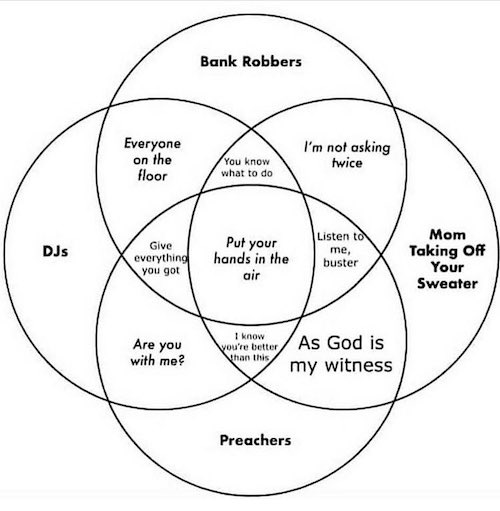Ch. 2 Probability

Sections covered: 2.1, 2.2, 2.3, 2.5

(2.4 will be covered later in the semester)

2.1 Sample Spaces and Events

Humor2.3 Counting Techniques

R

Factorial

##  120

Combinations

“5 choose 2” = choose 2 items out of 5

choose(5, 2)
##  10

Permutations

There is no built-in function to calculate permutations. You can multiply the number of combinations by k!.

Ex. Number of permutations of size 2 that can be formed from 5 distinct items:

##  20

You can create your own function to do this:

perm <- function(n, k) {choose(n,k)*factorial(k)}

perm(5,2)
##  20

2.5 Independence

Skip everything before “The Multiplication Rule” on p. 86 (until we return to conditional probability later in the semester)